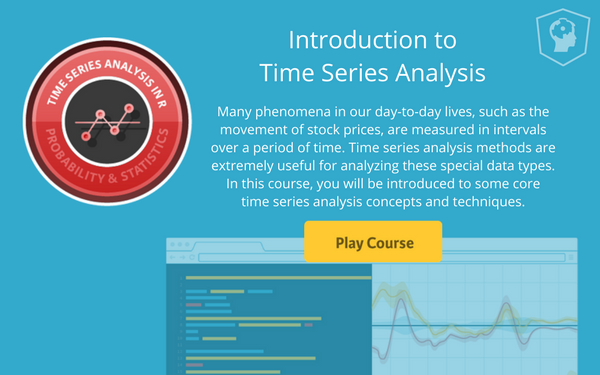Official Blog
r programming
+1

# New Course: Introduction to Time Series Analysis

Learn all about our newest time series course: Introduction to Time Series Analysis!

We just launched our newest time series course - Introduction to Time Series Analysis by David S. Matteson. Many phenomena in our day-to-day lives, such as the movement of stock prices, are measured in intervals over a period of time. Time series analysis methods are extremely useful for analyzing these special data types. In this course, you will be introduced to some core time series analysis concepts and techniques. Ready to get started?

Introduction to Time Series Analysis features 58 interactive exercises that combine high-quality video, in-browser coding, and gamification for an engaging learning experience that will make you a master at time series analysis!What you'll learn

The first chapter of this course gives you insights on how to organize and visualize time series data in R. You will learn several simplifying assumptions that are widely used in time series analysis and common characteristics of financial time series.

Next, you will conduct some trend spotting, and learn the white noise (WN) model, the random walk (RW) model, and the definition of stationary processes.

In the third chapter, you will review the correlation coefficient, use it to compare two time series, and also apply it to compare a time series with its past, as an autocorrelation. You will discover the autocorrelation function (ACF) and practice estimating and visualizing autocorrelations for time series data.

Next, you will learn the autoregressive (AR) model and several of its basic properties. You will also practice simulating and estimating the AR model in R, and compare the AR model with the random walk (RW) model. In the final chapter, you will learn the simple moving average (MA) model and several of its basic properties. You will also practice simulating and estimating the MA model in R, and compare the MA model with the autoregressive (AR) model.